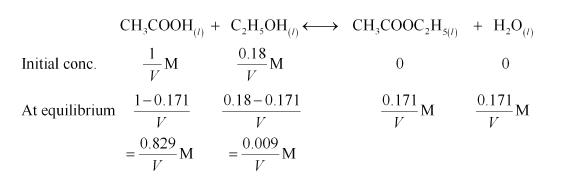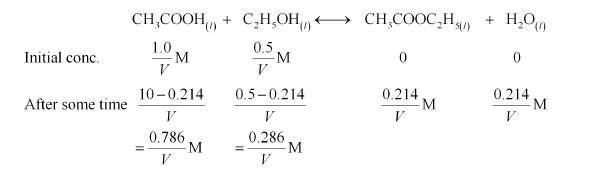# Ethyl acetate is formed by the reaction between ethanol and acetic acid and the equilibrium is represented as:Question:

Ethyl acetate is formed by the reaction between ethanol and acetic acid and the equilibrium is represented as:

$\mathrm{CH}_{3} \mathrm{COOH}(\mathrm{I})+\mathrm{C}_{2} \mathrm{H}_{5} \mathrm{OH}(\mathrm{I}) \longleftrightarrow \mathrm{CH}_{3} \mathrm{COOC}_{2} \mathrm{H}_{5}(\mathrm{I})+\mathrm{H}_{2} \mathrm{O}(\mathrm{l})$

(i) Write the concentration ratio (reaction quotient), Qc, for this reaction (note: water is not in excess and is not a solvent in this reaction)

(ii) At 293 K, if one starts with 1.00 mol of acetic acid and 0.18 mol of ethanol, there is 0.171 mol of ethyl acetate in the final equilibrium mixture. Calculate the equilibrium constant.

(iii) Starting with 0.5 mol of ethanol and 1.0 mol of acetic acid and maintaining it at 293 K, 0.214 mol of ethyl acetate is found after sometime. Has equilibrium been reached?

Solution:

(i) Reaction quotient, $Q_{\mathrm{C}}=\frac{\left[\mathrm{CH}_{3} \mathrm{COOC}_{2} \mathrm{H}_{5}\right]\left[\mathrm{H}_{2} \mathrm{O}\right]}{\left[\mathrm{CH}_{3} \mathrm{COOH}\right]\left[\mathrm{C}_{2} \mathrm{H}_{5} \mathrm{OH}\right]}$

(ii) Let the volume of the reaction mixture be $V$. Also, here we will consider that water is a solvent and is present in excess.

The given reaction is:Therefore, equilibrium constant for the given reaction is:

$K_{\mathrm{C}}=\frac{\left[\mathrm{CH}_{3} \mathrm{COOC}_{2} \mathrm{H}_{5}\right]\left[\mathrm{H}_{2} \mathrm{O}\right]}{\left[\mathrm{CH}_{3} \mathrm{COOH}\right]\left[\mathrm{C}_{2} \mathrm{H}_{5} \mathrm{OH}\right]}$

$=\frac{\frac{0.171}{V} \times \frac{0.171}{V}}{\frac{0.829}{V} \times \frac{0.009}{V}}=3.919$

$=3.92($ approximately $)$

(iii) Let the volume of the reaction mixture be V.Therefore, the reaction quotient is,

$Q_{\mathrm{C}}=\frac{\left[\mathrm{CH}_{3} \mathrm{COOC}_{2} \mathrm{H}_{5}\right]\left[\mathrm{H}_{2} \mathrm{O}\right]}{\left[\mathrm{CH}_{3} \mathrm{COOH}\right]\left[\mathrm{C}_{2} \mathrm{H}_{5} \mathrm{OH}\right]}$

$=\frac{\frac{0.214}{V} \times \frac{0.214}{V}}{\frac{0.786}{V} \times \frac{0.286}{V}}$

$=0.2037$

$=0204$ (approximately)

Since \$Q_{\mathrm{C}}